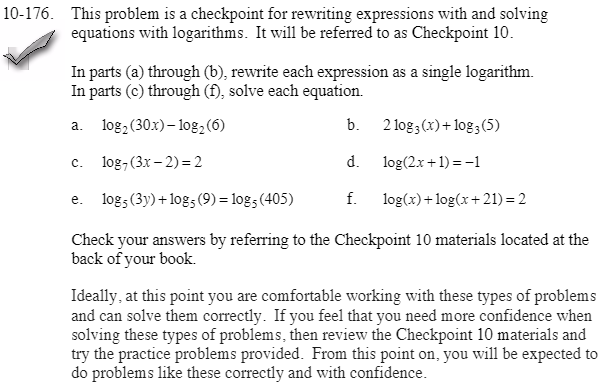Home > CCA2 > Chapter 10 > Lesson 10.3.2 > Problem10-176

10-176.
1. This problem is a checkpoint for rewriting expressions with and solving equations with logarithms. It will be referred to as Checkpoint 10. Homework Help ✎

2.In parts (a) through (b), rewrite each expression as a single logarithm. In parts (c) through (f), solve each equation.

1. log2(30x) − log2(6)

2. 2 log3(x) + log3(5)

3. log7(3x − 2) = 2

4. log(2x + 1) = −1

5. log5(3y) + log5(9) = log5(405)

6. log(x) + log(x + 21) = 2

3. Check your answers by referring to the Checkpoint 10 materials.

4. Ideally, at this point you are comfortable working with these types of problems and can solve them correctly. If you feel that you need more confidence when solving these types of problems, then review the Checkpoint 10 materials and try the practice problems provided. From this point on, you will be expected to do problems like these correctly and with confidence.Answers and extra practice for the Checkpoint problems are located in the back of your printed textbook or in the Reference Tab of your eBook. If you have an eBook for CCA2, login and then click the following link: Checkpoint 10: Rewriting Expressions and Solving Equations with Logarithms